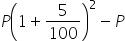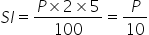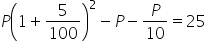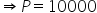Maths-
General
Easy

Question

# On what sum of money will the difference between the compound interest and simple interest for 2years be equal to Rs 25 if the rate of interest charged for both is 5% p.a.Hint:

## The correct answer is: Rs 10000

### Complete step by step solution:Let the principal amount = PIt is given that the rate of interest R = 5% and number of years T = 2 years.So, compound interest for 2 years =Simple  interest for 2 years =It is given that compound interest - simple interest = 25 RupeesThat is,Rupees.Hence the principal amount = Rs 10000#### With Turito Foundation.#### Get an Expert Advice From Turito.